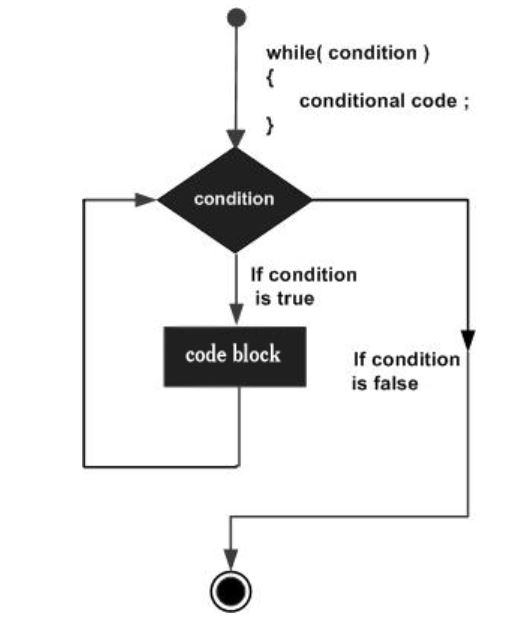×

Search anything:

# while loop in C++

#### C++ Software Engineering while loop loopGet this book -> Problems on Array: For Interviews and Competitive Programming

while loop is a most basic loop in C++. while loop has one control condition, and executes as long the condition is true. The condition of the loop is tested before the body of the loop is executed, hence it is called an entry-controlled loop.

Syntax :

``````While (condition)
{
statement(s);
Incrementation;
}
``````

NOTE : Key point of the while loop is that the loop might not ever run. When the condition is tested and the result is false, the loop body will be skipped and the first statement after the while loop will be executed.

### How while loop works

1. Condition is evaluated first.

2. If it returns true then the statements inside while loop execute.

3. Step 2 happens repeatedly until the condition returns false.

4. When condition returns false, the control comes out of loop and jumps to the next statement in the program after while loop.### Infinite while loop

A while loop that never stops is said to be the infinite while loop.
When we give the condition in such a way so that it never returns false, then the loops becomes infinite and repeats itself indefinitely.
An example of infinite while loop:
This loop would never end as I’m decrementing the value of i which is 1 so the condition i<=6 would never return false.

``````#include <iostream>
using namespace std;

int main()
{
int i=1;
while(i<=6)
{
cout<<"Value of variable i is: "<<i<<endl; i--;
}
return 0;
}
``````

### Example

``````#include <iostream>
using namespace std;

int main ()
{
// Local variable declaration:
int a = 10;

// while loop execution
while( a < 20 )
{
cout << "value of a: " << a << endl;
a++;
}

return 0;
}
``````

Output :

``````value of a: 10
value of a: 11
value of a: 12
value of a: 13
value of a: 14
value of a: 15
value of a: 16
value of a: 17
value of a: 18
value of a: 19
``````

### Question

Consider this C++ code:

``````#include <stdio.h>
void main()
{
int i = 0;
while (++i)
{
cout<<"H";
}
}
``````

#### What will be the output of the above code?

H is printed infinite times
H
compile time error
Varies
Condition given will never be false hence infinite loop.#### Harshita Sahai

Maintainer at OpenGenus | Previously Software Developer, Intern at OpenGenus (June to August 2019) | B.Tech in Information Technology from Guru Gobind Singh Indraprastha University (2017 to 2021)